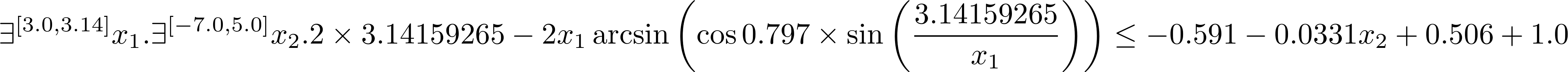dummy-link

7

6

4

5

# DReal.jlThis is a Julia wrapper for the dReal SMT solver. dReal allows you to answer satisfiability problems. That is, you can ask questions of the form: is there some assignment to my variables julia-observer-quote-cut-paste-0work,julia-observer-quote-cut-paste-1_work,julia-observer-quote-cut-paste-2work,..., that makes my formula over these variables true?_.

dReal also allows you to do non-linear, constrained, optimisation.

# Prerequisites

• Linux or OSX: DReal does not support Windows
• libstdc++6: In Ubuntu, please do the following install to install it.  sudo add-apt-repository --yes ppa:ubuntu-toolchain-r/test # needed for 12.04 sudo apt-get update sudo apt-get install libstdc++6 

# Installation

You can easily install DReal from a Julia repl with

Pkg.update()
Pkg.add("DReal")


DReal can then be loaded with:

using DReal


# Getting Started

To ask is there some Integer x and some Integer y such that x > 2 and y < 10 and x + 2*y ==7, y ou could write:

using DReal
x = Var(Int, "x")
y = Var(Int)
add!((x > 2) & (y < 10) & (x + 2*y == 7))
is_satisfiable()


The function Var(Int,"x") creates an integer variable named x. You can ommit the name and it will be automatically generated for you; for the most part you won't need to see the name. x will have type Ex{Int}, and when we apply functions to variables we also get back typed Ex{T} values. For instance x+2y will also have type Ex{Int}, but the main constraint (x > 2) & (y < 10) & (x + 2*y == 7) will have type Ex{Bool}, i.e. it is a proposition.

Use add! to assert that any proposition Ex{Bool} value must be true. We then use is_satisfiable to solve the system to determine whether there is any assignment to our variables x and y that can makes the expression x > 2 & y < 10 & x + 2*y == 7 true.

## Real Valued Arithmetic

Similarly to the previous example, we can use create models using Real (Float64) variables:

x = Var(Float64,-100.0,100.0)
y = Var(Float64,-100.0,100.0)
add!((x^2 + y^2 > 4) & (x^3 + y < 5))
x,y = model(x,y)


This example also shows how to extract a model. A model is an assignment of values to variables that makes the model satisfiable. Use model, and any variable used in the system to extract relevant variables in a model. Note: model only makes sense when the system is satisfiable, otherwise it will throw an error.

## Nonlinear Arithmetic

The feature which distinguishes dReal in comparison to other SMT solvers is its powerful support for nonlinear real arithmetic.

Consider an example which slighly modifies a formula from the Flyspeck project benchmarks, taken from the dReal homepage.x1 = Var(Float64, 3.0, 3.14)
x2 = Var(Float64, -7.0, 5.0)
c = (2π - 2*x1*asin(cos(0.797) * sin(π/x1))) <= (-0.591 - 0.0331 *x2 + 0.506 + 1.0)
add!(c)
is_satisfiable() # false


# Boolean Logic

dReal supports boolean operators: And (&), Or (|), Not (!), implies (implies or → (\rightarrow)) and if-then-else (ifelse). Bi­implications are represented using equality ==. The following example shows how to solve a simple set of Boolean constraints.

a = Var(Bool)
b = Var(Bool)
add!(a → b) # \rightarrow - equivalent to implies(a,b)
a,b =  model(a,b)